# Lines of Symmetry

##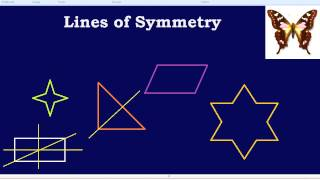By Bespoke education

How to find lines of symmetry.# [4.G.3-1.0] Lines of Symmetry - Common Core Standard

##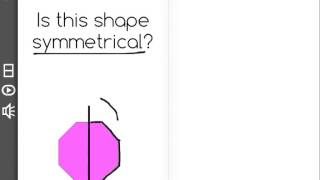By Freckle education

Recognize a line of symmetry for a two-dimensional figure as a line across the figure such that the figure can be folded along the line into matching parts.##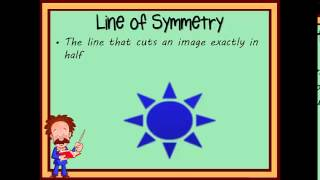By Brooke Wert

Recognize a line of symmetry for a two-dimensional figure as a line across the figure such that the figure can be folded along the line into matching parts.# Symmetry of two-dimensional shapes

##By Khan Academy

Sal solves the following problem: Two of the points that define a certain quadrilateral are (0,9) and (3,4). The quadrilateral has reflective symmetry over the line y=3-x. Draw and classify the quadrilateral.# Lines of Symmetry | MathHelp.com

##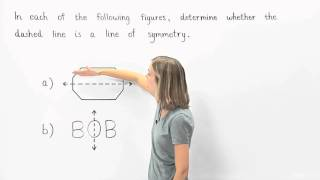By MathHelp.com# Elementary Algebra | MathHelp.com

##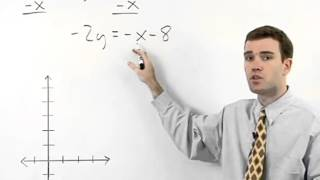By MathHelp.com

This lesson covers lines of symmetry. Students learn that a line of symmetry divides a figure into two parts, each of which is the mirror image of the other. In other words, if one side of a given figure is flipped over a line of symmetry, it will line up exactly with the other side. Students also learn that if a given figure is symmetric, then it has at least one line of symmetry. Students are then asked to determine if given figures are symmetric, and if so, draw all lines of symmetry.# Symmetry of two-dimensional shapes

##By Khan Academy

Sal solves the following problem: Two of the points that define a certain quadrilateral are (-4,-2) and (0,5). The quadrilateral has aï¿½ï¿½ï¿½ï¿½ï¿½ï¿½ï¿½ï¿½ï¿½ï¿½ï¿½ï¿½reflective symmetry over the lines y=x/2 andï¿½ï¿½ï¿½ï¿½ï¿½ï¿½ï¿½ï¿½ï¿½ï¿½ï¿½ï¿½y=-2x + 5.ï¿½ï¿½ï¿½ï¿½ï¿½ï¿½ï¿½ï¿½ï¿½ï¿½ï¿½ï¿½Draw and classify the quadrilateral.# Flipping the Classroom

##By Alyssa Balboni

Recognize a line of symmetry for a two-dimensional figure as a line across the figure such that the figure can be folded along the line into matching parts# Lines of Symmetry | MathHelp.com

##By MathHelp.com

MathHelp.com is great for teaching mathematics with custom math courses for Pre-Algebra, Algebra 1, Geometry, Algebra 2, and more. Perfect for homeschooling or for any student who needs lots of extra help in math.# Intercepts and Symmetry

##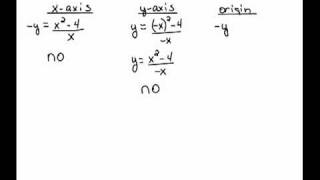By mathlablady1

Students will learn how to identify intercepts and test for symmetry in this video.# Symmetry and periodicity of trigonometric functions

##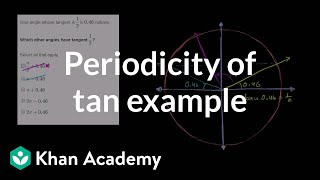By Khan Academy

Sal solves a problem by considering the periodicity of the tangent function.# Symmetry and periodicity of trigonometric functions

##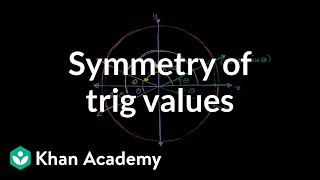By Khan Academy

Sal finds several trigonometric identities for sine and cosine by considering horizontal and vertical symmetries of the unit circle.# Symmetry and periodicity of trigonometric functions

##By Khan Academy

Sal finds several trigonometric identities for tangent by considering horizontal and vertical symmetries of the unit circle.# Symmetry and periodicity of trigonometric functions

##By Khan Academy

Sal finds trigonometric identities for sine and cosine by considering angle rotations on the unit circle.##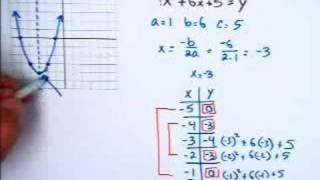By Marc Whitaker

Instructor uses a white board to model graphing quadratic equations. Examples show using quadratic equations in standard form to determine the line of symmetry, create a table of values, and graph the quadratic equation by using these values.# Symmetry of two-dimensional shapes

##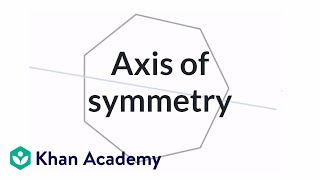By Khan Academy

Sal introduces the concept of an axis of symmetry.,1332# Symmetry and Symmetrical Figures

##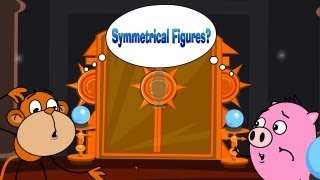By Magicpathashala

What is Symmetry# Symmetry of two-dimensional shapes

##By Khan Academy

Sal checks whether various figure are symmetrical under a 180 degrees rotation.# Introduction to Symmetry

##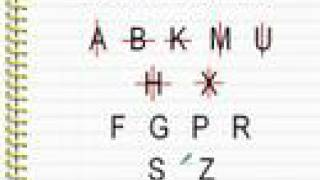By mathwithlarry

Introductory lesson concerning symmetry. Instructor Larry explains the general idea of symmetry and gives examples with letters in this clip. The instructor uses computer software for demonstration.# Grade 4 Math - Symmetry 1

##By Lumos Learning

Using the Lumos Study Programs, parents and educators can reinforce the classroom learning experience for children and help them succeed at school and on the standardized tests. Lumos books, dvd, eLearning and tutoring are used by leading schools, libraries and thousands of parents to supplement classroom learning and improve student achievement in the standardized tests.